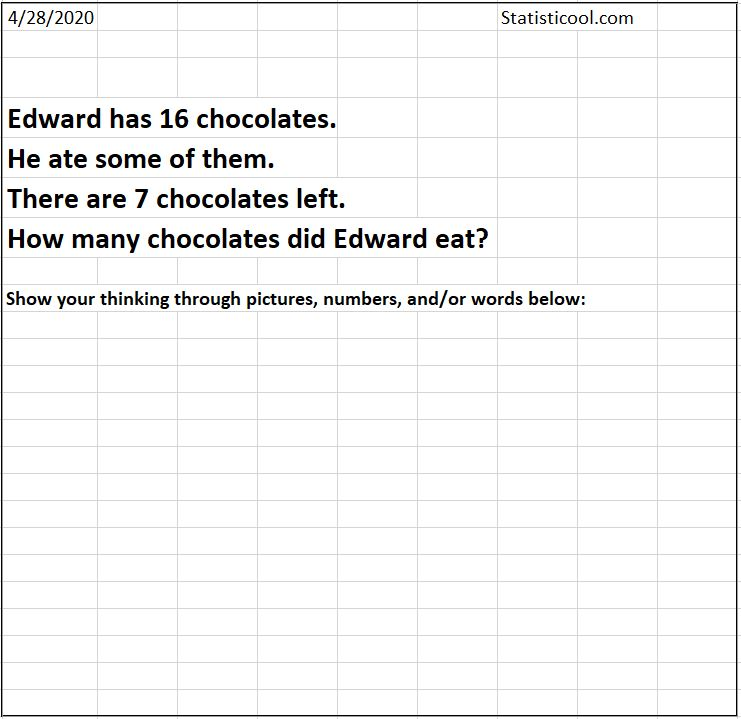# Subtraction Story

4/28/20

With the recent Coronavirus Disease 2019 (COVID-19), many children are at home because schools are closed. Education and fun, however, should not stop. Here is a spreadsheet I created that gets kids practicing subtraction and addition with subtraction stories.

In the spreadsheet, there is a column that randomly selects from several hundred male and female names.

There are columns for the numbers 0 through 20 with their English equivalents. This is because I have the spreadsheet randomly choose if it will display the actual number or the English equivalent in the subtraction stories. If you want to use numbers larger than 20, you will need to add on to these columns. Numbers are randomly selected from the range 2 to 20. I didn't allow 0 or 1 to be selected because it made the problems too easy, as well as increased the minor programming logic I'd need to add to account for singular words and other math and grammar special cases.

There are columns that are used to randomly select a subject, as well as the present and past tense verbs relating to this subject. Note that I tried to include some funny, gross, or entertaining examples that kids might like.The spreadsheet actually creates three subtraction stories. In each subtraction story the student needs to solve for the unknown x. The first subtraction story is of the "10-x=3" type. The second subtraction story is of the "10-3=x" type. The third subtraction story is of the "x-4=3" type. There are probability parameters that you can set for stories 1 and 2 that will make the spreadsheet randomly select from the three subtraction stories with your specified probabilities. Note, the probability of selecting the third subtraction story is just calculated as 1-(probability story 1 + probability story 2).

I set the Print Area, that is, the area that will be printed out when you click Print, to be the area with the words in the outlined box. This way, you can just open up the spreadsheet and print. You can also press F9 to refresh the random numbers and generate a new subtraction story.